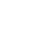# Use Sets with Ord Typeclass instances

Published 5 years ago
Updated 3 years ago

In Purescript, we can use set data structures using Purescript-Set, but many of its functions come with an Ord typeclass constraint. In this lesson, we'll learn how to understand the typeclass constraints on a function and how to create instances of typeclasses.

[00:00] Let's start with that blank file with the main, import Prelude, and so solve for F, and log hello sailor. Say we're working some kind of data type coords, and it's constructed with two integers. Then we can use this to create an empty set.

[00:15] We can say set is equal to empty. Here, we'll use the set A. Let's try to create a new set by inserting an element into it. We can say set two is equal to insert, where insert is the function for set A, and we're going to try a coords(1,1), and then set.

[00:37] Once we do this, we'll get an error. This error says that there's no ord instance for coords. For now, we should just comment this out.

[00:45] How do we go about implementing ord for coords? If we look at OM-Pursuit, we'll actually find that E equal A is the requirement for an ord.

[00:54] We first need to start off by defining an equal instance. Let's do instance equal coords, equal and coords, where equal and pattern match on AX, AY, and then BX, BY. This is going to be that if AX is equal to BX and AY is equal to BY, then that's an equal instance. That works, just matching X of both and Y of both.

[01:26] Now that we have an equal instance, we can actually test this out. Let's do a log show. This is just shorthand for log and show. Then we'll do the equal of coords(1,1) and coords(1,1).

[01:37] When we run this, we'll see that this is true. In PureScript, we can actually derive the instance for equal from the compiler. We can do derive instance equal coords, equal coords, and this will still work. The way ord works is that it has a single function, compare, which takes an A with order, and then takes another A to return an ordering.

[02:06] An ordering is a data type that's defined by less than, equal, and greater than. We can go look at that here. If we do log show of compare one and two, then when we print this out, we'll see less than. If we do log show of compare two and one, we'll see greater than.

[02:24] If we do log show of compare two and two, then we get equal. The orderings themselves actually have an ordering also. We can do log show of compare less than and equal, and this will return to less than. Using this knowledge, we should be able to define ord for coords.

[02:42] We'll do instance ord coords, ord coords, where compare and then pattern matching on AX, AY and coords BX, BY is equal to compare on the inner elements. Compare AX and BX, and then compare AX, BY. Then we can log out the comparison. Log show compare of coords(1,1), coords(1,2).

[03:19] When this prints out, we'll see that it's greater than. This is confusing, but we should remember this is the comparison of the orderings. What's actually going on here is it's comparing equal for the X element and then less than on the Y element, which printed out, will give us greater than, because equal comes after less than.

[03:40] Unfortunately, this implementation doesn't work because less than and less than compared to each other will be equal, and the same will happen for greater than and greater than. Ord is in the type class where the compiler can less us derive instances, so we don't actually need to do this manually.

[03:54] We can instead just do derive instance ord coords, ord coords, and this will work like we expect. The difference here being that, instead of doing naively the comparison on the compares, it actually does compare everything in order correctly, so we get less than for (1,1) compared to (1,2).

[04:16] Now that we have an instance for ord, we can actually go back and actually use this insert. Let's just pick some properties of our set. We do can log show, and use member for sets to find out if a given element is actually a part of the set.

[04:30] We'll use this with coords(1,1), and we'll do this on the original set, and again for the set number two. When we print this, we'll see that it's false for the first and true for the second. Let's try adding another set so that set three will be insert coords(1,2) into set two.

[04:53] We can inspect properties of this. We know that (1,1) will be part of set three, and we can inspect properties of this. We can know that (1,1) should be in set three, and then (1,2) should be in set three. When we print this out, we'll see that both conditions are true.

[05:12] This is how type classes work in PureScript, where a given method might have a type class constraint for a given type. For that given type, we need to create an instance of that type class. By creating instances of the type class, we gain access to functions that are defined for the type class.Justin Wooinstructor
~ 5 years ago

If you want to read more about type classes in Purescript, check out the section in Purescript by Example!